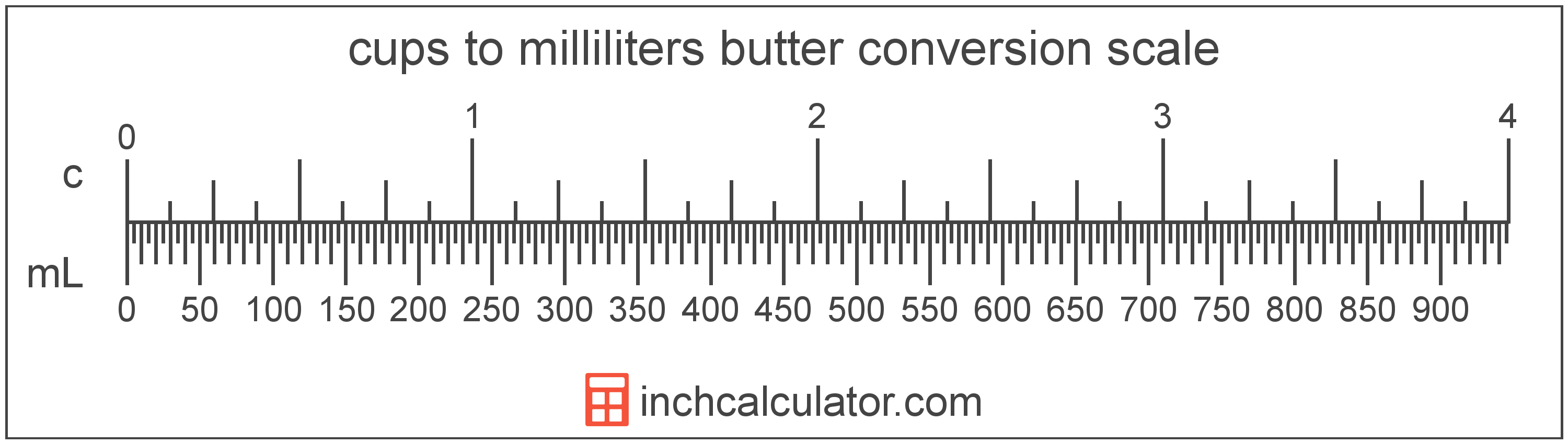# Convert Milliliters of Butter to Cups

Enter the butter in milliliters below to get the value converted to cups.

Results in Cups:1 mL = 0.004227 c

Do you want to convert cups to milliliters?

## How to Convert Milliliters to Cups

To convert a measurement in milliliters to a measurement in cups, multiply the butter by the following conversion ratio: 0.004227 cups/milliliter.

Since one milliliter of butter is equal to 0.004227 cups, you can use this simple formula to convert:

cups = milliliters × 0.004227

The butter in cups is equal to the butter in milliliters multiplied by 0.004227.

For example, here's how to convert 500 milliliters to cups using the formula above.
cups = (500 mL × 0.004227) = 2.113376 c## What is a Milliliter?

Butter is sometimes measured by volume in milliliters. One stick of butter contains about 118.3 milliliters.

The milliliter is an SI unit of volume in the metric system. A milliliter is sometimes also referred to as a millilitre. Milliliters can be abbreviated as mL; for example, 1 milliliter can be written as 1 mL.

## What is a Cup?

One cup of butter is equal to 2 sticks, or 1/2 pound.

The cup is a US customary unit of volume. Cups can be abbreviated as c, and are also sometimes abbreviated as C. For example, 1 cup can be written as 1 c or 1 C.

## Milliliter to Cup Conversion Table

Table showing various milliliter measurements converted to cups.
Milliliters Cups
1 mL 0.004227 c
2 mL 0.008454 c
3 mL 0.01268 c
4 mL 0.016907 c
5 mL 0.021134 c
6 mL 0.025361 c
7 mL 0.029587 c
8 mL 0.033814 c
9 mL 0.038041 c
10 mL 0.042268 c
20 mL 0.084535 c
30 mL 0.126803 c
40 mL 0.16907 c
50 mL 0.211338 c
60 mL 0.253605 c
70 mL 0.295873 c
80 mL 0.33814 c
90 mL 0.380408 c
100 mL 0.422675 c
200 mL 0.845351 c
300 mL 1.268 c
400 mL 1.6907 c
500 mL 2.1134 c
600 mL 2.5361 c
700 mL 2.9587 c
800 mL 3.3814 c
900 mL 3.8041 c
1,000 mL 4.2268 c